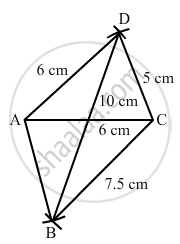Share

# Construct a Quadrilateral Abcd in Which Bc = 7.5 Cm, Ac = Ad = 6 Cm, Cd = 5 Cm and Bd = 10 Cm. - Mathematics

Course
ConceptConstructing a Quadrilateral When Two Diagonals and Three Sides Are Given

#### Question

Construct a quadrilateral ABCD in which BC = 7.5 cm, AC = AD = 6 cm, CD = 5 cm and BD = 10 cm.

#### Solution

Steps of construction:

Step I: Draw AC = 6 cm .

Step II: With A as the centre and radius 6 cm, draw an arc .

Step III : With C as the centre and radius 5 cm, draw an arc to intersect the arc drawn in Step II at D .

Step IV: With D as the centre and radius 10 cm, draw an arc on the other side of the line segment AC .

Step V : With C as the centre and radius 7 . 5 cm, draw an arc to intersect the arc drawn in Step IV at B .

Step VI: Join BA, DA, BC and CD to obtained the required quadrilateralIs there an error in this question or solution?

#### APPEARS IN

RD Sharma Solution for Mathematics for Class 8 by R D Sharma (2019-2020 Session) (2017 to Current)
Chapter 18: Practical Geometry (Constructions)
Ex. 18.2 | Q: 2 | Page no. 6
Solution Construct a Quadrilateral Abcd in Which Bc = 7.5 Cm, Ac = Ad = 6 Cm, Cd = 5 Cm and Bd = 10 Cm. Concept: Constructing a Quadrilateral - When Two Diagonals and Three Sides Are Given.
S